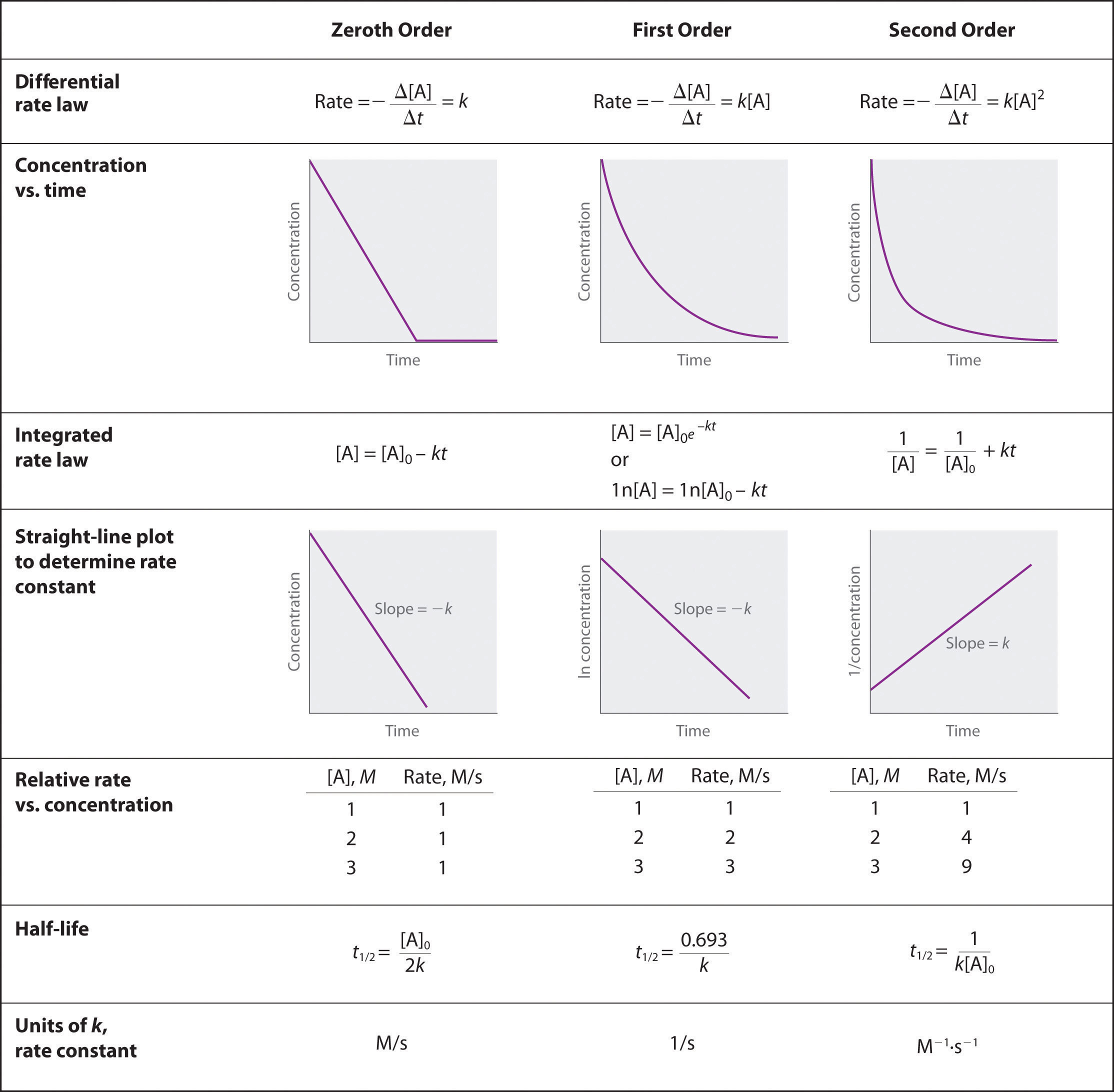Crystal

HS-PS1-4

# Reaction Order Study Guide

Order of reaction is the sum of the power of concentration of reactants written in rate law expression, and it can be an integer or fraction.

## INTRODUCTION

Reactions such as rusting take a lot of time to occur, while a neutralization reaction, i.e., when an acid reacts with a base, takes almost no time. So how fast or slow a reaction is, is called the rate of reaction, and the rate of reaction depends on the order. You must be wondering what reaction order is in chemistry? Let’s understand.

### REACTION ORDER

Order of reaction is the sum of the power of concentration of reactants written in rate law expression. Let’s understand in simple words. The rate of a reaction depends or sometimes doesn’t depend on the concentration of reactants.

Reaction rate = is the change in the amount of a reactant or product per unit time.

So, the reaction order is the relationship between the concentrations of species and the rate of a reaction. The order of reaction can be any integer or fraction. It can be found out only experimentally.

### ZERO-ORDER REACTIONS:

``````                                 A → B
``````

Here, the rate is independent of the concentration of the reactant, which is expressed as [A].

``````                             Rate =  k[A]0
``````

The power of [A] is 0, and hence the order of the reaction is zero. This means the rate of reaction won’t change even if we increase or decrease the concentration of the reactant.Source

### FIRST-ORDER REACTIONS:

``````                                A → B
``````

Here, the rate of reaction is proportional to the concentration of A. This means as [A] increases, the rate of reaction increases too and vice versa.

``````                            Rate = k [A]
``````

Since the power of [A] is 1, the order of the reaction is 1. The rate depends linearly on the concentration of reactant here. The rate of reaction is the slope of the graph.

### SECOND-ORDER REACTION:

``````                              A + B → C
``````

Here, the rate is expressed as k [A] [B], which means the rate will be dependent on both of the reactants A and B. If we increase or decrease the concentration of any of these, then the rate will change.

The rate is proportional to [A], and the order for A is 1, and the same is for B. But the overall order is the sum of orders with respect to both the reactants, so it will be 2.

## CONCLUSION:

• Order of reaction is the sum of the power of concentration of reactants written in rate law expression.
• It can be an integer or fraction.

### FAQs:

1. What is the order of a reaction?

Order of reaction is the sum of the power of concentration of reactants written in rate law expression.

2. Can the order of reaction be -½?

Yes, the order of the reaction can be an integer or fraction.

3. For Rate = k [A], what is the order of reaction?

Since the power of concentration of reactant is 1, the reaction is of the first order.

We hope you enjoyed studying this lesson and learned something cool about Reaction Order! Join our Discord community to get any questions you may have answered and to engage with other students just like you! Don't forget to download our App to experience our fun VR classrooms - we promise it makes studying much more fun! 😎

## SOURCES:

1. Reaction Order: https://www.ck12.org/c/chemistry/reaction-order/lesson/Order-of-Reaction-CHEM/?referrer=concept_details. Accessed 8th March 2022.
2. Reaction Order: https://chem.libretexts.org/Bookshelves/Physical_and_Theoretical_Chemistry_Textbook_Maps/Supplemental_Modules_(Physical_and_Theoretical_Chemistry)/Kinetics/03%3A_Rate_Laws/3.03%3A_The_Rate_Law/3.3.03%3A_Reaction_Order. Accessed 8th March 2022.